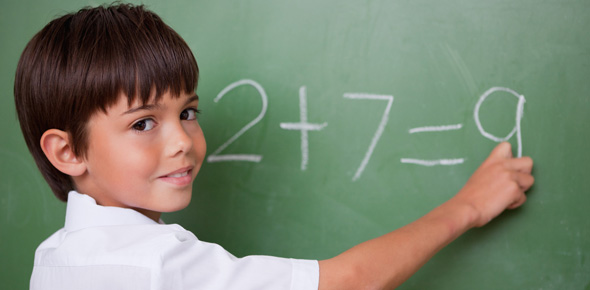5 Questions | Total Attempts: 17SettingsRelated Topics
• 1.
Count number of apples.  How many apples?
• 2.
Circle each group of ten squares.  Then write the number of groups of ten squares.
• 3.
Count the dots on the dominoes.  How many dots were all together?
• 4.
What number is missing to solve these problems?
1. 2+3 = ____
2. 3+____ = 8
• 5.
Which is the correct number sentence for this picture?
• A.

2+6 = 8

• B.

5+3 = 8

• C.

4+4 = 8